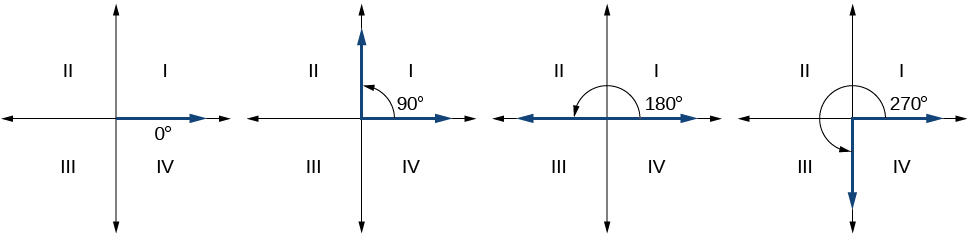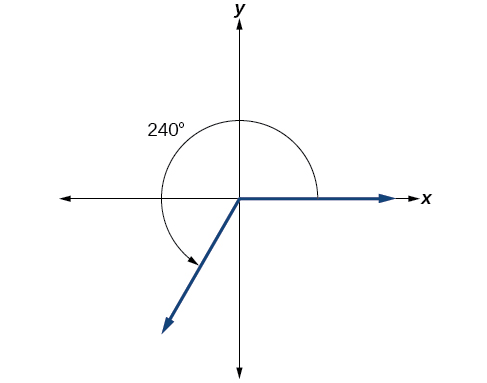# 7.1 Angles  (Page 2/29)

 Page 2 / 29

Drawing an angle in standard position always starts the same way—draw the initial side along the positive x -axis. To place the terminal side of the angle, we must calculate the fraction of a full rotation the angle represents. We do that by dividing the angle measure in degrees by $\text{\hspace{0.17em}}360°.\text{\hspace{0.17em}}$ For example, to draw a $\text{\hspace{0.17em}}90°\text{\hspace{0.17em}}$ angle, we calculate that $\text{\hspace{0.17em}}\frac{90°}{360°}=\frac{1}{4}.\text{\hspace{0.17em}}$ So, the terminal side will be one-fourth of the way around the circle, moving counterclockwise from the positive x -axis. To draw a $\text{\hspace{0.17em}}360°$ angle, we calculate that $\text{\hspace{0.17em}}\frac{360°}{360°}=1.\text{\hspace{0.17em}}$ So the terminal side will be 1 complete rotation around the circle, moving counterclockwise from the positive x -axis. In this case, the initial side and the terminal side overlap. See [link] .

Since we define an angle in standard position    by its terminal side, we have a special type of angle whose terminal side lies on an axis, a quadrantal angle . This type of angle can have a measure of $\text{0°,}\text{\hspace{0.17em}}\text{90°,}\text{\hspace{0.17em}}\text{180°,}\text{\hspace{0.17em}}\text{270°,}$ or $\text{\hspace{0.17em}}\text{360°}.\text{\hspace{0.17em}}$ See [link] .Quadrantal angles have a terminal side that lies along an axis. Examples are shown.

## Quadrantal angles

An angle is a quadrantal angle    if its terminal side lies on an axis, including $\text{0°,}\text{\hspace{0.17em}}\text{90°,}\text{\hspace{0.17em}}\text{180°,}\text{\hspace{0.17em}}\text{270°,}$ or $\text{\hspace{0.17em}}\text{360°}.$

Given an angle measure in degrees, draw the angle in standard position.

1. Express the angle measure as a fraction of $\text{\hspace{0.17em}}\text{360°}.$
2. Reduce the fraction to simplest form.
3. Draw an angle that contains that same fraction of the circle, beginning on the positive x -axis and moving counterclockwise for positive angles and clockwise for negative angles.

## Drawing an angle in standard position measured in degrees

1. Sketch an angle of $\text{\hspace{0.17em}}30°\text{\hspace{0.17em}}$ in standard position.
2. Sketch an angle of $\text{\hspace{0.17em}}-135°\text{\hspace{0.17em}}$ in standard position.
1. Divide the angle measure by $\text{\hspace{0.17em}}360°.$

$\frac{30°}{360°}=\frac{1}{12}$

To rewrite the fraction in a more familiar fraction, we can recognize that

$\frac{1}{12}=\frac{1}{3}\left(\frac{1}{4}\right)$

One-twelfth equals one-third of a quarter, so by dividing a quarter rotation into thirds, we can sketch a line at $\text{\hspace{0.17em}}30°,$ as in [link] .

2. Divide the angle measure by $\text{\hspace{0.17em}}360°.$

$\frac{-135°}{360°}=-\frac{3}{8}$

In this case, we can recognize that

$-\frac{3}{8}=-\frac{3}{2}\left(\frac{1}{4}\right)$

Negative three-eighths is one and one-half times a quarter, so we place a line by moving clockwise one full quarter and one-half of another quarter, as in [link] .

Show an angle of $\text{\hspace{0.17em}}240°\text{\hspace{0.17em}}$ on a circle in standard position.## Converting between degrees and radians

Dividing a circle into 360 parts is an arbitrary choice, although it creates the familiar degree measurement. We may choose other ways to divide a circle. To find another unit, think of the process of drawing a circle. Imagine that you stop before the circle is completed. The portion that you drew is referred to as an arc. An arc may be a portion of a full circle, a full circle, or more than a full circle, represented by more than one full rotation. The length of the arc around an entire circle is called the circumference of that circle.

The circumference of a circle is $\text{\hspace{0.17em}}C=2\pi r.\text{\hspace{0.17em}}$ If we divide both sides of this equation by $\text{\hspace{0.17em}}r,$ we create the ratio of the circumference, which is always $\text{\hspace{0.17em}}2\pi ,$ to the radius, regardless of the length of the radius. So the circumference of any circle is $\text{\hspace{0.17em}}2\pi \approx 6.28\text{\hspace{0.17em}}$ times the length of the radius. That means that if we took a string as long as the radius and used it to measure consecutive lengths around the circumference, there would be room for six full string-lengths and a little more than a quarter of a seventh, as shown in [link] .

#### Questions & Answers

A laser rangefinder is locked on a comet approaching Earth. The distance g(x), in kilometers, of the comet after x days, for x in the interval 0 to 30 days, is given by g(x)=250,000csc(π30x). Graph g(x) on the interval [0, 35]. Evaluate g(5)  and interpret the information. What is the minimum distance between the comet and Earth? When does this occur? To which constant in the equation does this correspond? Find and discuss the meaning of any vertical asymptotes.
Kaitlyn Reply
The sequence is {1,-1,1-1.....} has
amit Reply
circular region of radious
Kainat Reply
how can we solve this problem
Joel Reply
Sin(A+B) = sinBcosA+cosBsinA
Eseka Reply
Prove it
Eseka
Please prove it
Eseka
hi
Joel
June needs 45 gallons of punch. 2 different coolers. Bigger cooler is 5 times as large as smaller cooler. How many gallons in each cooler?
Arleathia Reply
7.5 and 37.5
Nando
find the sum of 28th term of the AP 3+10+17+---------
Prince Reply
I think you should say "28 terms" instead of "28th term"
Vedant
the 28th term is 175
Nando
192
Kenneth
if sequence sn is a such that sn>0 for all n and lim sn=0than prove that lim (s1 s2............ sn) ke hole power n =n
SANDESH Reply
write down the polynomial function with root 1/3,2,-3 with solution
Gift Reply
if A and B are subspaces of V prove that (A+B)/B=A/(A-B)
Pream Reply
write down the value of each of the following in surd form a)cos(-65°) b)sin(-180°)c)tan(225°)d)tan(135°)
Oroke Reply
Prove that (sinA/1-cosA - 1-cosA/sinA) (cosA/1-sinA - 1-sinA/cosA) = 4
kiruba Reply
what is the answer to dividing negative index
Morosi Reply
In a triangle ABC prove that. (b+c)cosA+(c+a)cosB+(a+b)cisC=a+b+c.
Shivam Reply
give me the waec 2019 questions
Aaron Reply

### Read also:

#### Get the best Algebra and trigonometry course in your pocket!

Source:  OpenStax, Algebra and trigonometry. OpenStax CNX. Nov 14, 2016 Download for free at https://legacy.cnx.org/content/col11758/1.6
Google Play and the Google Play logo are trademarks of Google Inc.

Notification Switch

Would you like to follow the 'Algebra and trigonometry' conversation and receive update notifications?ByByByBy Anonymous UserBy